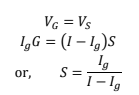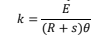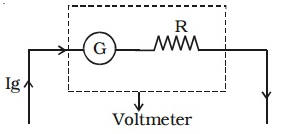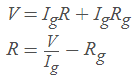Galvanometer and its conversion to Ammeter and VoltmeterGalvanometer

• A galvanometer is a low resistance device connected in series with the circuit. It is generally used for the following purposes:

• To detect the small current present in the circuit.
• To measure the small current in the circuit.
• To find the direction of current in the circuit.

A moving coil galvanometer consists of a rectangular coil of insulated copper wire wounded on a soft iron core placed between two strong pole pieces of the magnet. The pole piece of the magnet provides the magnetic field. This coil is suspended from a phosphor bronze. The two ends of the coil are connected to the two terminals which are used to connect to the circuit. When the current is passed through the coil in the magnetic field it experiences torque. So, this coil deflects from its equilibrium position. A needle is attached with the coil which also rotates with the coil. The deflection produced in the coil is proportional to the current passed through the coil. The needle is allowed for its maximum deflection of 90°. This overall arrangement is packed in a wooden or plastic box. The position of zero to maximum deflection is divided into appropriate range.

Since the galvanometer has low resistance, when it is connected in series it does not change the equivalent resistance of the circuit.

Conversion of galvanometer into ammeterAn ammeter is a device having low resistance used for measuring large electric currents in the circuits. Since the resistance is small, it does not affect the magnitude of the current when connected in series to the circuit. So, it is put in series with the circuit in which the current is to be measured.

A galvanometer can detect presence of small currents only. Thus, to measure large currents flowing through a circuit, we need an ammeter of appropriate range. For this, galvanometer is converted into an ammeter by connecting a low resistance called shunt resistance in parallel to the galvanometer.

The shunt connected in the circuit has following benefits

a. To convert a galvanometer into an ammeter.

b. It prevents the galvanometer from damage.

c. The equivalent resistance of the circuit decreases and the current increases.

Consider a galvanometer having resistance G and the maximum current that flow through the galvanometer when it shows the maximum deflection is Ig. Since the shunt is in parallel with the galvanometer resistance, potential across the galvanometer is equal to the potential across the shunt.Here the maximum current that can flow through the galvanometer when it shows maximum deflection Ig is

calculated using the equation, Ig = nk, where n is the number of divisions on the galvanometer and k is the figure of

merit of galvanometer. The figure of merit of a galvanometer is the current required in producing a unit deflection in

the scale of the galvanometer. Generally, figure of merit is represented by k and is given by the equation,Where E is the E.M.F the cell and θ is the deflection produced with resistance R connected across the cell.

Conversion of galvanometer in voltmeterA voltmeter is a high resistance device used to measure the potential difference between two points in an electrical circuit. Since voltmeter consists of high resistance, it should be connected in series with the circuit.

To convert galvanometer into voltmeter, we should connect high resistance in series with the galvanometer. The high resistance connected in series with the galvanometer to convert it into voltmeter is called multiplier. Since the resistance of the multiplier is greater than the resistance of the galvanometer, large part of the potential is dropped across the multiplier and remaining potential is dropped across the galvanometer. Let Rg  be the resistance of galvanometer and be the maximum current that can flow through the galvanometer for full scale deflection. Since the multiplier is connected in series, total potential difference is equal to the sum of potential across the galvanometer and the multiplier.This is the value of the multiplier that must be connected in series with the galvanometer. Here most of the potential is dropped across the multiplier and the potential is only drop across the galvanometer. This multiplier protects the galvanometer from damage due to flow of large current when connected across the large potential.